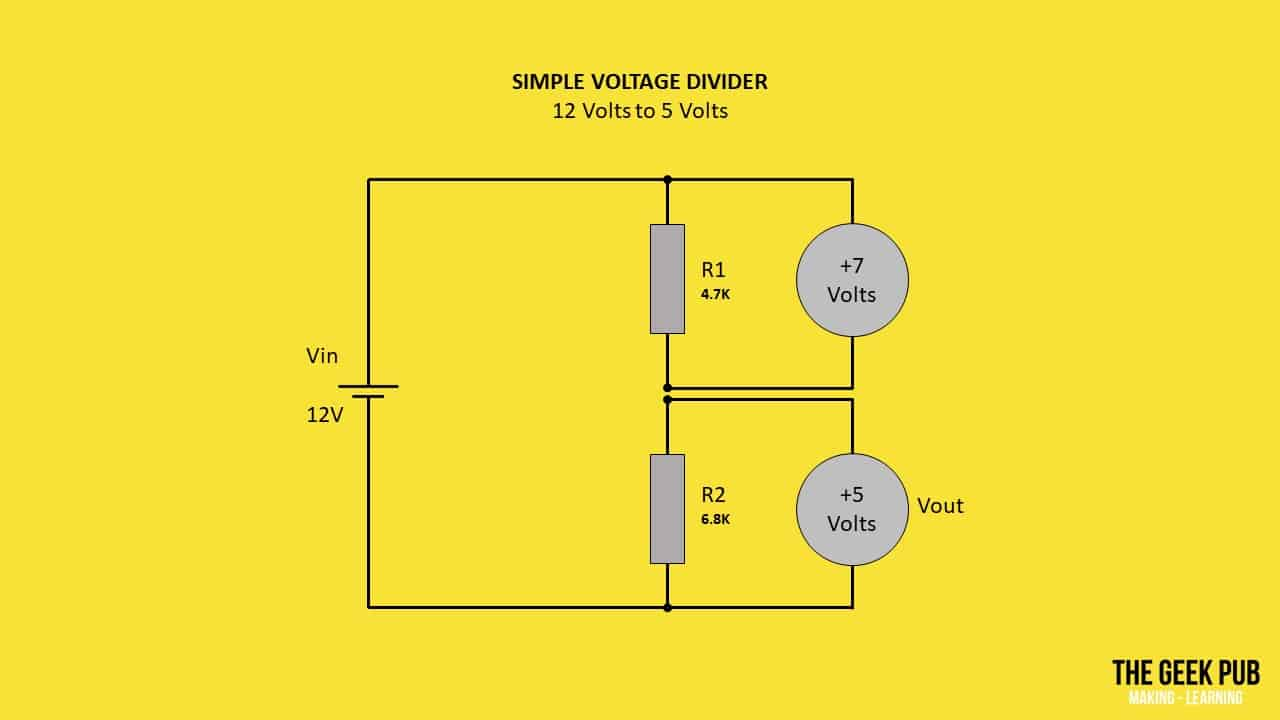At its most basic, a voltage divider is a circuit that divides higher voltages into lower voltages using a pair of resistors. This can be a complex task for beginners that don’t yet understand all of the basics or how to use the voltage divider formula. That’s why we put together this handy voltage divider calculator that you can use to calculate the resistance needed for your voltage divider circuits. All you need to do is enter any three of the values below and the missing one will be calculated automatically. It couldn’t be simpler!

## Voltage Divider Calculator

 Input Voltage (Vin): Volt Resistance 1 (R1) Ohm Kilo Ohm Mega Ohm Resistance 2 (R2) Ohm Kilo Ohm Mega Ohm Output Voltage (Vout): Volt

Our voltage divider calculator works using the standard voltage divider formula: Vout= (Vin x R2) / (R1 + R2)

Let’s break this down a little bit to make it simpler to understand:

• Vin is the input voltage of your power supply or battery
• R1 is the resistance of the 1st resistor in Ohms
• R2 is the resistance of the 2nd resistor in Ohms
• Vout is the output voltage from the voltage divider

You can see in this schematic illustration that using two resistors (R1=4.7K Ohm, R2= 6.8K Ohm) that we can create a 5 volt output from 12 volts.  This voltage divider converts 12 volt to 5 volt:You can use the above voltage divider calculator to calculate any of these values quickly.  We’ve also put together a table of the most common voltage dividers you can pick from:

 Resistor Combination Use 4.7 kΩ and 6.8 kΩ 12V to 5V 4.7 kΩ and 3.9 kΩ 9V to 5V 3.6 kΩ and 9.1 kΩ 12V to 3.3V 3.3 kΩ and 5.7 kΩ 9V to 3.3V

You should try popping these values in the voltage divider calculator just so you can see how the outputs work! It’s a great way to get familiar with it (it will help you understand the voltage divider formula).

## Voltage Divider Considerations

There are few things you should keep in mind when using the voltage divider calculator and formula. The formula, nor the calculator will size the components for you or regulate this voltage!

• Voltage regulation: Voltage dividers do not regulate voltage. If the input voltage is not stable and fluctuates, so will the outputs!  If you need a solid reliable power source you may want to consider adding a voltage regulator to your circuit.
• Proper sizing: Be sure to properly size the resistors to handle the load you plan to run across this circuit.  Most resistors are only capable of 1/4 or 1/2 watt! Something a lot people forget. You’ll need bigger resistors for bigger loads.  You may want to check out our resistor tutorial.  And let’s be honest, you shouldn’t be using a voltage divider to power large loads. It’s not efficient and there are better options!
• Potentiometers: Don’t overlook the potentiometers when building a voltage divider. With a potentiometer you can adjust the resistance and dial in your voltage divider.  This can be especially handy when prototyping something and the exact design direction is not yet decided.